# Basic Trigonometric Functions

The basic trigonometric functions can be defined in terms of a right triangle. For the angle θ at one apex of the right triangle the functions can be defined by: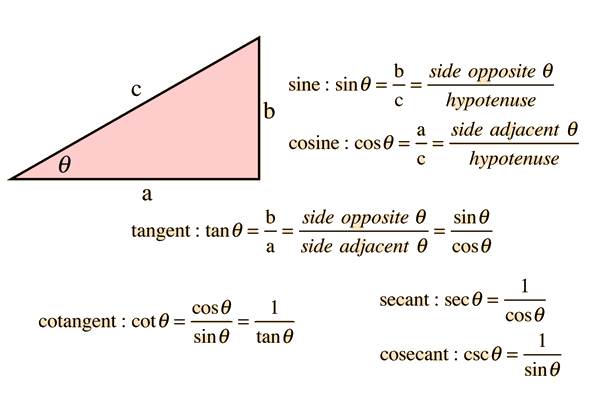### Basic triangle geometry

Index

 HyperPhysics****HyperMath*****Trigonometry R Nave
Go Back

# The Arctangent Problem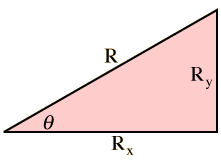In the right triangle shown, the angle can be calculated from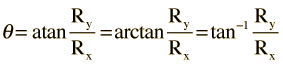(several notations for the same function.) A common problem which arises in vector addition when the components of the resultant vector are found is that the arctan function in calculators and computer languages cannot distinguish the quadrant of the angle.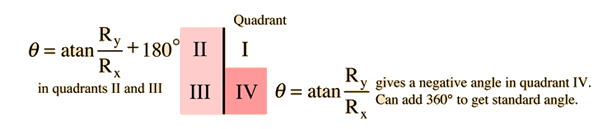Right triangle geometry More detail on arctan problem
Index

 HyperPhysics****HyperMath*****Trigonometry R Nave
Go Back

# Arctan Problem Details

Care must be taken when calculating angles from coordinates because of the arctangent problem.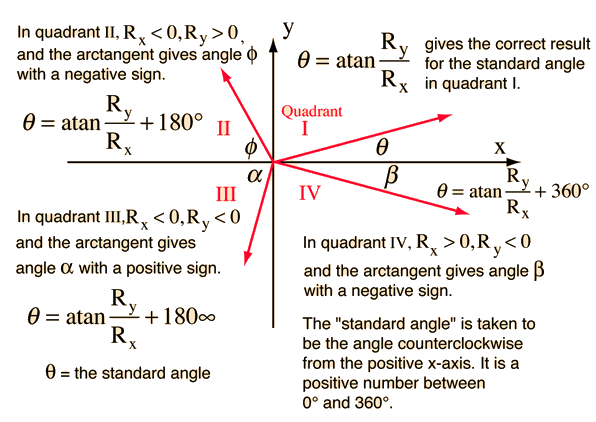If Rx = and Ry = then θ = °
Index

 HyperPhysics****HyperMath*****Trigonometry R Nave
Go Back